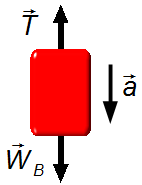Solved Problem on DynamicsPortuguêsEnglish

An Atwood machine has masses, of mA = 6.25 kg and mB = 6.75 kg, connected by a weightless cord, through a pulley frictionless. Find:
a) The acceleration of the system;
b) The tension in the rope connecting the masses;
c) The tension in the rope that holds the system to the ceiling.
Assume the free-fall acceleration g = 10 m/s2.Problem data
• mass of body A:    mA = 6.25 kg;
• mass of body B:    mB = 6.75 kg;
• free-fall acceleration:    g = 10 m/s2.
Problem diagram

Since the mass of block B is greater than the mass of block A, block B drops while block A rises, as the cord has a negligible mass and the pulley is frictionless, the acceleration is the same for the whole system.
We consider a system of reference oriented positively, in the direction of block B moves downward, in the same direction of free-fall acceleration.
As the rope has a negligible mass, it only transmits the tension from one side to the other side of the pulley between blocks (figure 1).figure 1
Solution

Drawing a free-body diagram for each block and applying Newton's Second Law, we have
$\bbox[#99CCFF,10px] {\vec{F}=m\vec{a}}$
Body A
• $$\vec{T}$$ : tension force on the rope;
• $${\vec{W}}_{\text{A}}$$ : weight of block A.
$T-W_{\text{A}}=m_{\text{A}}a \tag{I}$figure 2
The weight of body A is given by
$W_{\text{A}}=m_{\text{A}}g$
substituting the expression (II) in (I), we have
$T-m_{\text{A}}g=m_{\text{A}}a \tag{III}$
Body B
• $$\vec{T}$$ : tension on the cord;
• $${\vec{W}}_{\text{B}}$$ : weight of block B.
$W_{\text{B}}-T=m_{\text{B}}a \tag{IV}$
The weight of body B is given byfigure 3
$W_{\text{B}}=m_{\text{B}}g \tag{V}$
substituting the expression (V) in (IV), we have
$m_{\text{B}}g-T=m_{\text{B}}a\tag{VI}$
Expressions (II) and (IV) can be written as a system of linear equations with two variables (a and T)
$\left\{\begin{matrix} \;T-m_{\text{A}}g=m_{\text{A}}a\\ \;m_{\text{B}}g-T=m_{\text{B}}a \end{matrix}\right.\$
substituting the values given in the problem and adding the two equations
$\left\{\begin{matrix} \;T-6.25 \times 10=6.25 a\\ \;6.75 \times 10-T=6.75 a \end{matrix}\right.\\ \frac{\left\{\begin{matrix} \;\cancel{T}-62.5=6.25 a\\ \;67.5-\cancel{T}=6.75 a \end{matrix}\right.}{5=13 a}\\ a=\frac{5}{13}\\$
$\bbox[#FFCCCC,10px] {a=0.38\;\text{m/s}^{2}}$

b) Substituting the value found in item (a) in the first (or second equation of the system) we obtain the tension in the rope
$T-62.5=6.25\times0.38\\ T-62.5=2.38\\ T=2.38+62.5$
$\bbox[#FFCCCC,10px] {T=64.88\;\text{N}}$

c) Since the pulley distributes the tension equally in the rope on both sides of the pulley, the tension in the rope that supports the system in the roof will be double (figure 1)
$2T=2\times64.88$
$\bbox[#FFCCCC,10px] {2T=129.76\;\text{N}}$